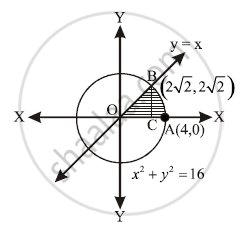# Find the area of the sector of a circle bounded by the circle x^2 + y^2 = 16 and the line y = x in the ftrst quadrant. - Mathematics and Statistics

Find the area of the sector of a circle bounded by the circle x2 + y2 = 16 and the line y = x in the ftrst quadrant.

#### SolutionGiven that x2 + y2 =16 ...(i)

y=x ...........(ii)

By equation (i) & (ii)

x=+-2sqrt2

y=+-2sqrt2

But required area in first quadrant

x= y=2sqrt2

From dig. area = Area of Δ OBC + Area of region CABC

=int_o^(2sqrt2)x dx+int_(2sqrt2)^4sqrt(16-x^2)dx

=1/2 [x^2]_0^(2sqrt2)+[x/2sqrt(16-x^2)+16/2 sin^-1 (x/4)]_sqrt2^4

=4+8 xxpi/2-4-8xxpi/4=2pi sq.units

Concept: Area of the Region Bounded by a Curve and a Line
Is there an error in this question or solution?